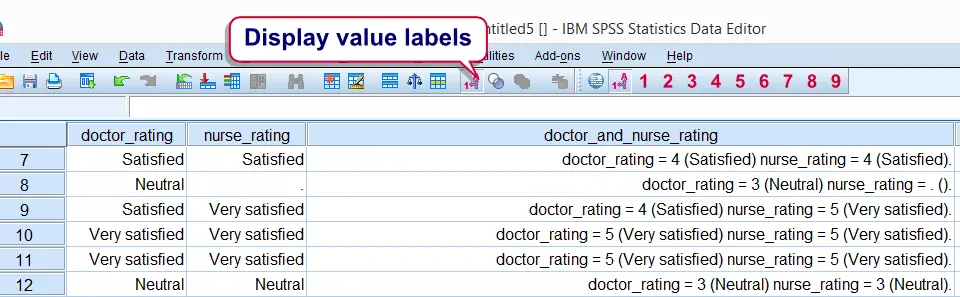SPSS TUTORIALS BASICS ANOVA REGRESSION FACTOR CORRELATION

# Combine Categorical Variables

Many easy options have been proposed for combining the values of categorical variables in SPSS. However, the real information is usually in the value labels instead of the values. This tutorial proposes a simple trick for combining categorical variables and automatically applying correct value labels to the result.

## SPSS Combine Categorical Variables Example

You may follow along by downloading and opening hospital.sav. Now say we'd like to combine “doctor_rating” and “nurse_rating” (near the end of the file). The result is shown in the screenshot below. Note that all variables are numeric with proper value labels applied to them.## SPSS Combine Categorical Variables Syntax

We first present the syntax that does the trick. Next, we'll point out how it how to easily use it on other data files.

*1. Declare new tmp string variable.

string tmp(a1000).

*2. Combine values and value labels of doctor_rating and nurse_rating into tmp string variable.

compute tmp = concat(
"doctor_rating = ",string(doctor_rating,f1)," (",rtrim(valuelabels(doctor_rating)),") ",
"nurse_rating = ",string(nurse_rating,f1)," (",rtrim(valuelabels(nurse_rating)),") "
).

*3. Convert string variable into numeric.

autorecode tmp
/into doctor_and_nurse_rating.

*4. Delete tmp string variable.

delete variables tmp.

*5. Optionally, apply variable label to end result.

variable labels doctor_and_nurse_rating 'Combination of doctor_rating and nurse_rating'.

## SPSS Combine Categorical Variables - Other Data

We realize that many readers may find this syntax too difficult to rewrite for their own data files. So instead of rewriting it, just copy and paste it and make three basic adjustments before running it:

1. replace “doctor_rating” by the name of the first variable you'd like to combine. Note that you can do so by using the ctrl + h shortkey.
2. replace “nurse_rating” by the name of the second variable you'd like to combine.
3. replace “doctor_and_nurse_rating” by the variable name you'd like to use for the final result.

## SPSS Combine Categorical Variables - System Missing Values

You may have noticed that the value labels of the combined variable don't look very nice if system missing values are present in the original values. An example of such a value label is doctor_rating = 3 (Neutral) nurse_rating = . (). A nicer result can be obtained without changing the basic syntax for combining categorical variables. Prior to running this syntax, simply RECODE system missing values. Use a value that's not yet present in the original variables and apply a value label to it. The syntax below shows how to do so.

*1. Recode system missing into value that doesn't occur in variable yet.

recode doctor_rating nurse_rating (sysmis = 7).

*2. Apply value label to new value.

add value labels doctor_rating nurse_rating 7 'System missing'.

*3. Proceed with remaining syntax from here.

After doing so, the resulting value label will look as follows: doctor_rating = 3 (Neutral) nurse_rating = 7 (System missing). Further, note that the syntax we used made a couple of assumptions. Most real world data will satisfy those. We'll walk through them below.

## SPSS Combine Categorical Variables - Assumptions

• Although the syntax combines two variables, it can be expanded to incorporate three or more variables.
• It is assumed that all values in the original variables consist of single digits. If two or three digit values are present, replace `f1` by `n2` or `n3`.The `n3` format left pads numbers with zeroes and thus keeps their alphabetical order equal to their numerical order.

Those who'd like a closer look at some of the commands and functions we combined in this tutorial may want to consult string variables, STRING function, VALUELABEL, CONCAT, RTRIM and AUTORECODE.

# Tell us what you think!

*Required field. Your comment will show up after approval from a moderator.

# THIS TUTORIAL HAS 24 COMMENTS:

•### By setu on January 7th, 2021

hi, I want to merge 2 categorical variables named mother's education level and father's education level into one variable named parental education. if both are no education named illiterate, then.... how can I do this?

•### By Ruben Geert van den Berg on January 7th, 2021

Hi Setu!

How are these variables coded? And what is "parental education" if mother is high and father is low?

•### By Maria on July 21st, 2021

Hello!

I have a question. I want to merge a categorical variable (Likert scale) but then keep all the ones that answered one together. In other words not sum them but keep the categories...just merged together...is this possible?

•### By Ruben Geert van den Berg on July 22nd, 2021

Hi Maria!

If I understand correctly, we covered this in SPSS - Merge Categories of Categorical Variable.

Hope that helps!

SPSS tutorials